JMSLTM Numerical Library 7.2.0
com.imsl.stat

## Class NormTwoSample

• All Implemented Interfaces:
Serializable, Cloneable

```public class NormTwoSample
extends Object
implements Serializable, Cloneable```
Computes statistics for mean and variance inferences using samples from two normal populations.

Class `NormTwoSample` computes statistics for making inferences about the means and variances of two normal populations, using independent samples in `x1` and `x2`. Missing values, that is, values equal to NaN (not a number), are excluded from the computations. For inferences concerning parameters of a single normal population, see class `NormOneSample`.

Let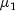and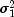be the mean and variance of the first population, and let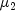and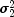be the corresponding quantities of the second population. The function contains test confidence intervals for difference in means, equality of variances, and the pooled variance.

The means and variances for the two samples are as follows: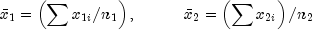and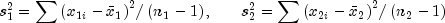The test that the difference in means equals a certain value, for example,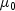, depends on whether or not the variances of the two populations can be considered equal. If the variances are equal and `meanHypothesis` equals 0, the test is the two-sample t-test, which is equivalent to an analysis-of-variance test. The pooled variance for the difference-in-means test is as follows: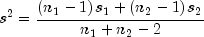The t statistic is as follows: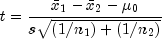Also, the confidence interval for the difference in means can be obtained by first assigning the unequal variances flag to false. This can be done by calling the `setUnequalVariances` method. The confidence interval can then be obtained by the `getLowerCIDiff` and `getUpperCIDiff` methods.

If the population variances are not equal, the ordinary t statistic does not have a t distribution and several approximate tests for the equality of means have been proposed. (See, for example, Anderson and Bancroft 1952, and Kendall and Stuart 1979.) One of the earliest tests devised for this situation is the Fisher-Behrens test, based on Fisher's concept of fiducial probability. A procedure used in the `getTTest`, `getLowerCIDiff` and `getUpperCIDiff` methods assuming unequal variances are specified is the Satterthwaite's procedure, as suggested by H.F. Smith and modified by F.E. Satterthwaite (Anderson and Bancroft 1952, p. 83). Use `setUnequalVariances` true to obtain results assuming unequal variances.

The test statistic is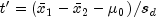where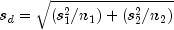Under the null hypothesis of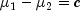, this quantity has an approximate t distribution with degrees of freedom `df`, given by the following equation: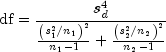The F statistic for testing the equality of variances is given by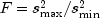, where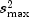is the larger of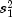and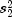. If the variances are equal, this quantity has an F distribution with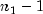and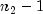degrees of freedom.

It is generally not recommended that the results of the F test be used to decide whether to use the regular t-test or the modified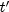on a single set of data. The modified(Satterthwaite's procedure) is the more conservative approach to use if there is doubt about the equality of the variances.

Example 1, Example 2, Serialized Form
• ### Constructor Summary

Constructors
Constructor and Description
```NormTwoSample(double[] x, double[] y)```
Constructor to compute statistics for mean and variance inferences using samples from two normal populations.
• ### Method Summary

Methods
Modifier and Type Method and Description
`void` `downdateX(double[] x)`
Removes the observations in `x` from the first sample.
`void` `downdateY(double[] y)`
Removes the observations in `y` from the second sample.
`double` `getChiSquaredTest()`
Returns the test statistic associated with the chi-squared test for common, or pooled, variances.
`int` `getChiSquaredTestDF()`
Returns the degrees of freedom associated with the chi-squared test for the common, or pooled, variances.
`double` `getChiSquaredTestP()`
Returns the probability of a larger chi-squared associated with the chi-squared test for common, or pooled, variances.
`double` `getDiffMean()`
Returns the difference in means, mean of `x` - mean of `y`.
`double` `getFTest()`
Returns the F test value of the F test for equality of variances.
`int` `getFTestDFdenominator()`
Returns the denominator degrees of freedom of the F test for equality of variances.
`int` `getFTestDFnumerator()`
Returns the numerator degrees of freedom of the F test for equality of variances.
`double` `getFTestP()`
Returns the probability of a larger F in absolute value for the F test for equality of variances, assuming equal variances.
`double` `getLowerCICommonVariance()`
Returns the lower confidence limits for the common, or pooled, variance.
`double` `getLowerCIDiff()`
Returns the lower confidence limit for the mean of the first population minus the mean of the second for equal or unequal variances depending on the value set by setUnequalVariances.
`double` `getLowerCIRatioVariance()`
Returns the approximate lower confidence limit for the ratio of the variance of the first population to the second.
`double` `getMeanX()`
Returns the mean of the first sample, `x`.
`double` `getMeanY()`
Returns the mean of the second sample, `y`.
`double` `getPooledVariance()`
Returns the Pooled variance for the two samples.
`double` `getStdDevX()`
Returns the standard deviation of the first sample.
`double` `getStdDevY()`
Returns the standard deviation of the second sample.
`double` `getTTest()`
Returns the test statistic for the Satterthwaite's approximation.
`double` `getTTestDF()`
Returns the degrees of freedom for the Satterthwaite's approximation for t-test for either equal or unequal variances, depending on the value set by `setUnequalVariances`.
`double` `getTTestP()`
Returns the approximate probability of a larger `t` for the Satterthwaite's approximation for equal or unequal variances.
`double` `getUpperCICommonVariance()`
Returns the upper confidence limits for the common, or pooled, variance.
`double` `getUpperCIDiff()`
Returns the upper confidence limit for the mean of the first population minus the mean of the second for equal or unequal variances depending on the value set by `setUnequalVariances`.
`double` `getUpperCIRatioVariance()`
Returns the approximate upper confidence limit for the ratio of the variance of the first population to the second.
`void` `setChiSquaredTestNull(double varianceHypothesisValue)`
Sets the null hypothesis value for the chi-squared test.
`void` `setConfidenceMean(double confidenceMean)`
Sets the confidence level (in percent) for a two-sided interval estimate of the mean of `x` - the mean of `y`, in percent.
`void` `setConfidenceVariance(double confidenceVariance)`
Sets the confidence level (in percent) for two-sided interval estimate of the variances.
`void` `setTTestNull(double meanHypothesis)`
Sets the Null hypothesis value for t-test for the mean.
`void` `setUnequalVariances(boolean eqVar)`
Specifies whether to return statistics based on equal or unequal variances.
`void` ```update(double[] x, double[] y)```
Concatenates samples x and y to the samples provided in the constructor.
`void` `updateX(double[] x)`
Concatenates the values in `x` to the first sample provided in the constructor.
`void` `updateY(double[] y)`
Concatenates the values in `y` to the second sample provided in the constructor.
• ### Methods inherited from class java.lang.Object

`clone, equals, finalize, getClass, hashCode, notify, notifyAll, toString, wait, wait, wait`
• ### Constructor Detail

• #### NormTwoSample

```public NormTwoSample(double[] x,
double[] y)```
Constructor to compute statistics for mean and variance inferences using samples from two normal populations.
Parameters:
`x` - is a `double` array containing the first sample.
`y` - is a `double` array containing the second sample.
• ### Method Detail

• #### downdateX

`public void downdateX(double[] x)`
Removes the observations in `x` from the first sample.
Parameters:
`x` - is a `double` array containing the values to remove from the first sample.
• #### downdateY

`public void downdateY(double[] y)`
Removes the observations in `y` from the second sample.
Parameters:
`y` - is a `double` array containing the values to remove from the second sample.
• #### getChiSquaredTest

`public double getChiSquaredTest()`
Returns the test statistic associated with the chi-squared test for common, or pooled, variances. The chi-squared test is a test of the hypothesis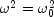where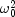is the null hypothesis value as described in `setChiSquaredTestNull`.
Returns:
a `double` containing the test statistic for the chi-squared test.
• #### getChiSquaredTestDF

`public int getChiSquaredTestDF()`
Returns the degrees of freedom associated with the chi-squared test for the common, or pooled, variances. The chi-squared test is a test of the hypothesiswhereis the null hypothesis value as described in `setChiSquaredTestNull`.
Returns:
an `int` containing the degrees of freedom for the chi-squared test.
• #### getChiSquaredTestP

`public double getChiSquaredTestP()`
Returns the probability of a larger chi-squared associated with the chi-squared test for common, or pooled, variances. The chi-squared test is a test of the hypothesiswhereis the null hypothesis value as described in `setChiSquaredTestNull`.
Returns:
a `double` containing the probability of a larger chi-squared for the chi-squared test for variances.
• #### getDiffMean

`public double getDiffMean()`
Returns the difference in means, mean of `x` - mean of `y`.
Returns:
a `double` containing the difference in mean.
• #### getFTest

`public double getFTest()`
Returns the F test value of the F test for equality of variances.
Returns:
a `double` containing the F test value of the F test for equality of variances.
• #### getFTestDFdenominator

`public int getFTestDFdenominator()`
Returns the denominator degrees of freedom of the F test for equality of variances.
Returns:
an `int` containing the denominator degrees of freedom.
• #### getFTestDFnumerator

`public int getFTestDFnumerator()`
Returns the numerator degrees of freedom of the F test for equality of variances.
Returns:
an `int` containing the numerator degrees of freedom.
• #### getFTestP

`public double getFTestP()`
Returns the probability of a larger F in absolute value for the F test for equality of variances, assuming equal variances.
Returns:
a `double` containing the probability of a larger F in absolute value, assuming equal variances.
• #### getLowerCICommonVariance

`public double getLowerCICommonVariance()`
Returns the lower confidence limits for the common, or pooled, variance.
Returns:
a `double` containing the lower confidence limits for the variance.
• #### getLowerCIDiff

`public double getLowerCIDiff()`
Returns the lower confidence limit for the mean of the first population minus the mean of the second for equal or unequal variances depending on the value set by setUnequalVariances. `setUnequalVariances`
Returns:
a `double` containing the lower confidence limit for the mean of the first sample minus the mean of the second sample.
• #### getLowerCIRatioVariance

`public double getLowerCIRatioVariance()`
Returns the approximate lower confidence limit for the ratio of the variance of the first population to the second.
Returns:
a `double` containing the approximate lower confidence limit variance.
• #### getMeanX

`public double getMeanX()`
Returns the mean of the first sample, `x`.
Returns:
a `double` containing the mean.
• #### getMeanY

`public double getMeanY()`
Returns the mean of the second sample, `y`.
Returns:
a `double` containing the mean.
• #### getPooledVariance

`public double getPooledVariance()`
Returns the Pooled variance for the two samples.
Returns:
a `double` containing the Pooled variance for the two samples.
• #### getStdDevX

`public double getStdDevX()`
Returns the standard deviation of the first sample.
Returns:
a `double` containing the standard deviation of the first sample.
• #### getStdDevY

`public double getStdDevY()`
Returns the standard deviation of the second sample.
Returns:
a `double` containing the standard deviation of the second sample.
• #### getTTest

`public double getTTest()`
Returns the test statistic for the Satterthwaite's approximation. The value returned will be based on assumption of equal or unequal variances based on the the value set by `setUnequalVariances`. `setUnequalVariances`
Returns:
a `double` containing the test statistic for the t-test.
• #### getTTestDF

`public double getTTestDF()`
Returns the degrees of freedom for the Satterthwaite's approximation for t-test for either equal or unequal variances, depending on the value set by `setUnequalVariances`. `setUnequalVariances`
Returns:
an `double` containing the degrees of freedom for the t-test.
• #### getTTestP

`public double getTTestP()`
Returns the approximate probability of a larger `t` for the Satterthwaite's approximation for equal or unequal variances. `setUnequalVariances`
Returns:
a `double` containing the probability for the t-test.
• #### getUpperCICommonVariance

`public double getUpperCICommonVariance()`
Returns the upper confidence limits for the common, or pooled, variance.
Returns:
a `double` containing the upper confidence limits for the variance.
• #### getUpperCIDiff

`public double getUpperCIDiff()`
Returns the upper confidence limit for the mean of the first population minus the mean of the second for equal or unequal variances depending on the value set by `setUnequalVariances`. `setUnequalVariances`
Returns:
a `double` containing the upper confidence limit for the mean of the first sample minus the mean of the second sample.
• #### getUpperCIRatioVariance

`public double getUpperCIRatioVariance()`
Returns the approximate upper confidence limit for the ratio of the variance of the first population to the second.
Returns:
a `double` containing the approximate upper confidence limit variance.
• #### setChiSquaredTestNull

`public void setChiSquaredTestNull(double varianceHypothesisValue)`
Sets the null hypothesis value for the chi-squared test. The default is 1.0.
Parameters:
`varianceHypothesisValue` - a `double` containing the null hypothesis value for the chi-squared test.
• #### setConfidenceMean

`public void setConfidenceMean(double confidenceMean)`
Sets the confidence level (in percent) for a two-sided interval estimate of the mean of `x` - the mean of `y`, in percent. Argument `confidenceMean` must be between 0.0 and 1.0 and is often 0.90, 0.95 or 0.99. For a one-sided confidence interval with confidence level c (at least 50 percent), set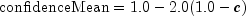. If the confidence mean is not specified, a 95-percent confidence interval is computed. Default: confidenceMean = .95
Parameters:
`confidenceMean` - `double` containing the confidence level of the mean.
• #### setConfidenceVariance

`public void setConfidenceVariance(double confidenceVariance)`
Sets the confidence level (in percent) for two-sided interval estimate of the variances. Under the assumption of equal variances, the pooled variance is used to obtain a two-sided `confidenceVariance` percent confidence interval for the common variance with `getLowerCICommonVariance` or `getUpperCICommonVariance`. Without making the assumption of equal variances, `setUnequalVariances` , the ratio of the variances is of interest. A two-sided `confidenceVariance` percent confidence interval for the ratio of the variance of the first sample to that of the second sample is given by the `getLowerCIRatioVariance` and `getUpperCIRatioVariance`. See `setUnequalVariances` and `getUpperCIRatioVariance`. The confidence intervals are symmetric in probability. Argument `confidenceVariance` must be between 0.0 and 1.0 and is often 0.90, 0.95 or 0.99. The default is 0.95.
Parameters:
`confidenceVariance` - `double` containing the confidence level of the variance.
• #### setTTestNull

`public void setTTestNull(double meanHypothesis)`
Sets the Null hypothesis value for t-test for the mean. `meanHypothesis`=0.0 by default.
Parameters:
`meanHypothesis` - `double` containing the hypothesis value.
• #### setUnequalVariances

`public void setUnequalVariances(boolean eqVar)`
Specifies whether to return statistics based on equal or unequal variances. The default is to return statistics for equal variances. if `eqVar` is True then statistics for unequal variances will be returned.
Parameters:
`eqVar` - a `boolean` containing a true or false value. A value of true will cause results for unequal variances to be returned. A value of false will cause results for equal variances to be returned.
• #### update

```public void update(double[] x,
double[] y)```
Concatenates samples x and y to the samples provided in the constructor.

This method updates the test results to include a new subset of the data. This is useful when the data is too large to fit into memory or when all of the data is not available at one time or location.

Parameters:
`x` - is a `double` array containing updates to the first sample.
`y` - is a `double` array containing updates to the second sample.
• #### updateX

`public void updateX(double[] x)`
Concatenates the values in `x` to the first sample provided in the constructor.

This method updates the test results to include a new subset of the data. This is useful when the data is too large to fit into memory or when all of the data is not available at one time or location.

Parameters:
`x` - is a `double` array containing updates for the first sample.
• #### updateY

`public void updateY(double[] y)`
Concatenates the values in `y` to the second sample provided in the constructor.

This method updates the test results to include a new subset of the data. This is useful when the data is too large to fit into memory or when all of the data is not available at one time or location.

Parameters:
`y` - is a `double` array containing updates for the second sample.
JMSLTM Numerical Library 7.2.0